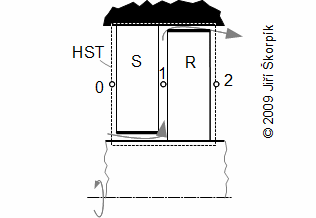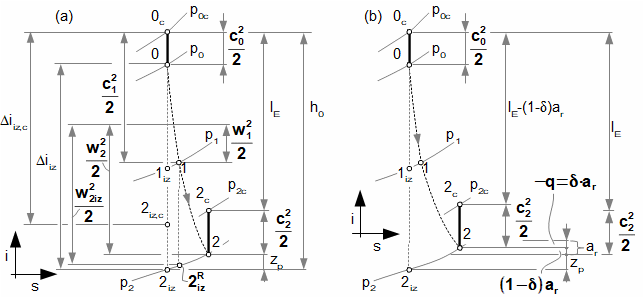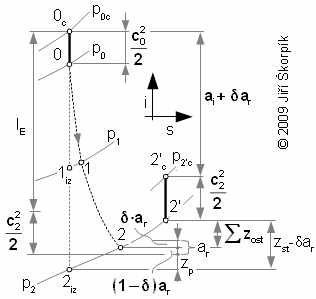RELATION BETWEEN SPECIFIC SHAFT WORK AND INTERNAL WORK OF TURBOMACHINE STAGE

i-s diagram of stage with taking into account rotor friction losses
Efficiency of stage
The article of online continued resource Transformační technologie; ISSN 1804-8293;
www.transformacni-technologie.cz; Copyright©Jiří Škorpík, 2006-2020. All rights reserved. This work was published without any linguistic and editorial revisions.
● 14. Relation between specific shaft work and internal work of turbomachine stage ●

Introduction

This article follows the article Essential equations of turbomachines and Energy balances of turbomachines. In these articles are defined quantities a specific shaft work lu and a specific internal work of turbomachine stage ai.

The specific internal work of stage is corresponded work of the working fluid inside stage and it is calculated from difference of stagnation states between the inlet and the exit of stage. The specific work shaft is corresponded work of the working fluid transformed to the torque of the shaft and it is calculated from a velocity triangels in front of and behind the rotor and from a friction between the rotor and the working fluid so called rotor friction losses:

Rotor friction losses

The specific shaft work of real machines is influenced by friction between the rotor and the working fluid so called rotor friction losses:1.318 Difference between work shaft and internal work of stage.HST volume of stage; r [m] radius; lu [J·kg-1] specific work shaft of stage on radius r(1); c [m·s-1] absolute velocity; w [m·s-1] relative velocity; u [m·s-1] circumference velocity; ar [J·kg-1] rotor friction losses of stage(2); lE [J·kg-1] specific work of working fluid during flow through rotor blade row without rotor friction losses; ai [J·kg-1] specific internal work of stage; A, B areas of development friction losses under friction between rotor and working fuid. S stator blade row; R rotor blade row.
(1)Remark
The specific shaft work can be changed along the lenght of blade. Also the pressure is changed along the lenght of blade under pressure gradient.
(2)Rotor friction losses
The friction is function of a construction of the stage, it is the biggest in stages with a disc rotor (e.g. the one-stage Laval turbine or radial stages), this friction is usually negligible for stages with drum rotors.

The rotor friction losses of the stage is a consumed work, which is transformed on heat. This friction heat heatings the working fluid in surrounding:

● 1 ●
● 14. Relation between specific shaft work and internal work of turbomachine stage ●2.934 Subdividing of heat flow from rotor friction.
δ [-] coefficient subdividing of heat flow from rotor friction; δ·ar [J·kg-1] heat flow to part of machine (heat extraction to surrounding); (1-δ)ar [J·kg-1] heat flow to working fluid.

From equation of specific internal work of turbomachine is evident heat δ·ar increases reajected heat to surroundings and heat (1-δ)ar increases internal heat at the exit of stage ue respective enthalpy ie. This decreases the specific internal work and the specific shaft work same, therefore for now be can lu=ai.

The conclusion of the previous paragraph was formulated on the assumption that the working fluid flow only through blade passage at development only the profile losses and rotor friction losses without the other losses of the stage. But the turbomachine stage is a classically engineering product, which usually is not perfectly, therefore there are other losses(3) inside stages also e.g. a portion of the working fluid can flow outside the blade passages (leaks, construction gap) etc.:3.1089 Example of flow through a leak of a turbine stage.
(3)Other losses of the stage
These losses are depend by type of a construction of the stage and its quality (inside one stage can be a few types of the other losses). More information is shown in the article 17. Losses in turbomachines.

In computing practice, the term spedific shaft lu means the internal work of the stage without the influence of other losses (perfectly tight stage and without losses occurring at the root and tips of the blades, we can also speak of a stage with infinitely long blades). The internal specific work of stage ai is then the actual specific work of stage including all losses (lu>ai).

i-s diagram of stage with taking into account rotor friction losses

For turbine stages be can constructed i-s diagram according the chapters Adiabatic expansion inside heat turbine, Polytropic expansion inside heat turbine:

● 2 ●
● 14. Relation between specific shaft work and internal work of turbomachine stage ●4.936 i-s diagram of heat turbine stage.
The figure shows expansion inside a reaction axial stage on a tested radius r. (a) case for ar<<lE – negligible influence of rotor friction losses(4); (b) case for ar>0. i [J·kg-1] specific enthalpy; s [J·kg-1·K-1] specific entropy; p [Pa] pressure; zp [J·kg-1] specific blade profile loss (friction between working fluid and surface of blades); q [J·kg-1] specific heat of working gas transfer with surroundings. Subscript iz denotes the state of the working fluid at exit of the blade rows for case isentropic process, subscript denotes c stagnation state.
(4)Remark
For the indication of the kinetic energy of the relative velocities in the i-s diagram can to help equation for the specific shaft work. From this equation is evident, the sum of the specific shaft work lE must be equal to the sum of the kinetic energy on the inlet of the stage and the difference of the kinetic energy of the relative velocities.

For stages of working machines be can constructed i-s diagram according the chapters Adiabatic compression inside compressor, Polytropic compression inside compressor:5.719 i-s diagram of compressor stage on radius r.
● 3 ●
● 14. Relation between specific shaft work and internal work of turbomachine stage ●

Total energy balance of stage

Total energy balance of the stage in i-s diagram shows all losses, enthalpy and the work of the working fluid at the exit for case ideal mixing:6.319 The specific internal work of a turbomachine stage on a tested radius r.zst [J·kg-1] total losses of stage. The other losses of stage increases enthalpy from state 2 on the state 2' at the exit.

If the other losses influences state of the working fluid inside core flow at exit first blade row then it can be influenced proces inside second blade row:7.947 Influence the other losses on the work shaft.zns [J·kg-1] specific loss through leaks of stator. This is case from Figure 5 (ar≈0 J·kg-1). The flow inside core of stage is influenced by leaks on the stator blade row in this case. The working fluid from seal of the stator increases enthalpy at the inlet rotor blade row.

The shape of i-s diagram of flow through blade row of the stage corresponds to construction of stage. The i-s diagrams axial and diagonal stages are shown in article 19. Design of axials turbomachine stages, and in article 20. Design of radials turbomachine stages are shown i-s diagrams of radial stages.

For cases turbomachines without casing the flow mass of the working fluid beside the stream-tube of rotor are not included to the other losses zost.

● 4 ●
● 14. Relation between specific shaft work and internal work of turbomachine stage ●

Efficiency of stage

Two efficiencies of the stage are defined because two work of the stage are defined:8.876 The specific shaft work efficiency and the thermodynamic efficiency of a turbine stage.
ηE [-] specific shaft work efficiency of turbine stage without other losses; e0 [J·kg-1] specific energy of working fluid at inlet of stage; (a) [J·kg-1] portion of specific kinetic energy of inlet velocity of working fluid, which is used inside stage(5); (b) [J·kg-1] portion of specific kinetic energy of exit velocity of working fluid, which is used inside next stage(6); ηst [-] internal (thermodynamic) efficiency of stage. Approximate is true c2,iz=c2.
(5)Remark
The value of the coefficient κ0 is in interval from 0 to 1. Usually it is required κ0=1. The requirement κ0<1 there are if the losses between a measuring point of the velocity c0 (e.g. the exit previous stage) and a beginning of the blade row are not a portion of the losses of the stage. So, the value of the coefficient κ0 is connected with definition of the boundary stage. For example, the boundary of the wind turbine stage a designer can define tightly in front of the rotor then vortex losses, which are developed between the inlet to the stream-tube and the rotor are not included in energy balance of the rotor and the value of the coefficient is κ0<1.
(6)Remark
The value of the coefficient κ2 is in interval from 0 to 1. For cases multi-stage turbomachines is κ2=1 (in these cases the kinetic energy of the working fluid at the exit of the stage is not considered as a loss), only for case the last of the stages or one-stage machines is used κ2=0.

For cases stages of working machines is usually defined the effective efficiency respectively isentropic efficiency of the stage. These efficiency are connected with static state of the working fliud at isentropic processes:9.356 The effective efficiency and isentropic efficiency of a working machine stage.
ηef [-] effective efficiency of working machine stage (usually specific shaft work is without other losses in this case); ηiz [-] isentropic efficiency of stage; ηiz, c [-] isentropic efficiency of stage in relation to stagnation state on exit of stage (for usually case c2=c2, iz is true ηiz, ciz).
The difference of the enthalpy of steam inside one stage of a steam turbine for isentropic process is 21,3 kJ·kg-1. The exit of the stage is the same as the velocity of steam at the inlet to the stage. The calculated blade profile losses of the stage are 3,3970 kJ·kg-1 (the rotor blade row is geometrically same as the stator blade row). The calculated internal work of stage is 16,0744 kJ·kg-1. Calculate work shaft efficiency and the internal efficiency of this stage. This stage is the first stage of a multi-stage steam turbine. The solution of this problem is shown in the Appendix 923.
Problem 1.923
● 5 ●
● 14. Relation between specific shaft work and internal work of turbomachine stage ●

Efficiency of group of stages

The sum of the energy balance all stages and the energy balance of other section of the turbine must be equal the energy balance of the stage section of the turbine as one entire:10.116 A multistage adiabatic expansion inside turbine.ηj [-] mean internal efficiency of individual stages; 1+f [-] reheat factor (coefficient of the re-usable heat, 1,02 to 1,04 according ); Δ [J·kg-1] re-usable heat of turbine; 1+f∞ [-] reheat factor for case heat turbine with infinite number of stages; z [-] numbers of stages. The efficiency of stage section of multi-stage turbines ηi e.g. multi-stage steam turbines is higher than ηj of individual stages. These equation are derived for the same the enthalpy difference for all stages and for adiabatic expansion. For better illustration is not drawn the absolute velocity c. The derivation of this equation is shown in the Appendix 116.

It is evident, a portion of the heat from the loss processes inside previous stage is used inside next stage during expansion. Only the heat from the loss processes inside last stage of the heat turbine are not used. The re-usable heat influences as well as flow through one stage, because a portion of stator losses be can use in the rotor etc.

In case multi-stage turbocompressors, the resulting compression is composed of several sub-compression, their number is equal number of the stages inside turbocompressor:11.121 An multistage compression inside turbocompressor.1+f [-] preheat factor (coefficient of additional losses); Δj [-] additional losses of one stage; 1+f∞ [-] preheat factor in theoretical case turbocompressor with infinite number of stages. The efficiency ηi of multi-stage turbocompressor is less than ηj. These equation are derived for the same the enthalpy difference for all stages and for adibatic compresion. For better illustration is not drawn the absolute velocity c. The derivation of these equations is shown in the Appendix 121.
● 6 ●
● 14. Relation between specific shaft work and internal work of turbomachine stage ●

It is evident, the internal losses of one turbocompressor stage reduces the internal efficiency of next stage. The additional losses influences as well as flow through one stage, because losses of rotor increases losses of stator etc.

The air with temperature 15 °C and pressure 0,1013 MPa enters to a turbocompressor, on exit of the turbocompressor the air has temperature 293 °C and pressure 0,802 MPa. Calculate work ai, aiz, internal efficiency ηi and factor 1+f. Number of stages of the turbocompressor is 12. Use simplification cp=const.. The solution of this problem is shown in the Appendix 122. This problem is published in .
Problem 2.122

References

1. KADRNOŽKA, Jaroslav. Tepelné turbíny a turbokompresory I, 2004. 1. vydání. Brno: Akademické nakladatelství CERM, s.r.o., ISBN 80-7204-346-3.